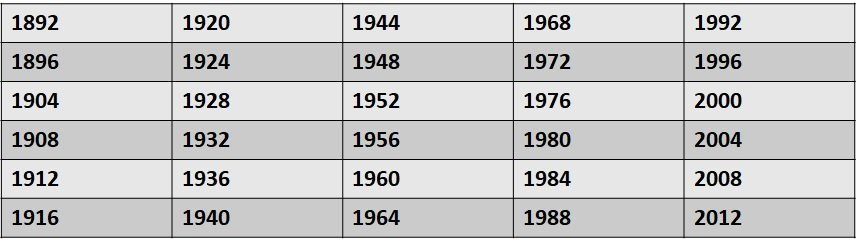Leap Year Program in ABAP is a mathematical expression based algorithm that is common across all other programming languages. In this article, we will discuss what is a leap year and how to write ABAP program to find if a year is a leap year or not.

## Introduction

Leap Year is a year that has 29 days in the month of February. It means 366 days in year. If a year is divisible by 100, then it must be also divisible by 400 to become a leap year. Also, if the year is not divisible by 100 then in case it is divisible by 4, then it is also a leap year.

Following are the past years that were leap year:Leap Year Program in ABAP Image Illustration

## ABAP Program

Following ABAP program will find a year is a leap year or not:

```PARAMETERS: p_date TYPE dats.
DATA : lv_date TYPE num4.
lv_date = p_date.
IF lv_date MOD 4 = 0.
IF lv_date MOD 100 = 0.
IF lv_date MOD 400 = 0.
WRITE : 'Leap Year '.
ELSE.
WRITE : 'Not a Leap Year '.
ENDIF.
ELSE.
WRITE : 'Leap Year '.
ENDIF.
ELSE.
WRITE : 'Not a Leap Year '.
ENDIF.
```

## Explanation

To understand this program, we will discuss each lines one by one:

1. Initially we have defined a parameter p_date of type DATS that is date type in ABAP. Also, we have defined a variable which will convert the date in a numeric date type using type conversion.
2. Now If a year is divisible by 4, 100 and 400 then it is a leap year. We have added IF-ELSE condition accordingly.

This site uses Akismet to reduce spam. Learn how your comment data is processed.

Translate »